Related Articles
Build a DFA to accept Binary strings that starts or ends with “01”
• Last Updated : 07 Nov, 2020

Given binary string str, the task is to build a DFA that accepts the string if the string either starts with “01” or ends with “01”.

Input: str = “010000”
Output: Accepted
Explanation:
The given string starts with “01”.

Input: str = “1100111”
Output: Not Accepted
Explanation:
The given string neither starts with nor ends with “01”.

DFA or Deterministic Finite Automata is a finite state machine that accepts a string(under some specific condition) if it reaches a final state, otherwise rejects it.
In DFA, there is no concept of memory, therefore we have to check the string character by character, beginning with the 0th character. The input set of characters for the problem is {0, 1}. For a DFA to be valid, there must a transition rule defined for each symbol of the input set at every state to a valid state. Therefore, the following steps are followed to design the DFA:

• In this case, the strings that start with 01 or end with 01 or both start with 01 and end with 01 should be acceptable.
• Make an initial state and transit its input alphabets, i.e, 0 and 1 to two different states.
• Check for acceptance of string after each transition to ignore errors.
• First, make DfA for minimum length string then go ahead step by step.
• Define Final State(s) according to the acceptance of string.

Step by Step Approach to design a DFA:

• Step 1: Make an initial state “A”. The minimum possible string is 01 which is acceptable. For this, make the transition of 0 from state “A” to state “B” and then make the transition of 1 from state “B” to state “C” and notice this state “C” as the final state.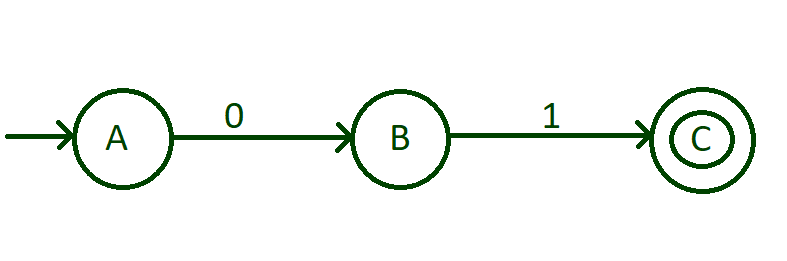• Step 2: Now, we have designed the DFA that start with 01. To accept all the strings that start with 01 like 011, 010, 010000, 01111, 010101000010001 etc, we need to put a self-loop of 0 and 1 to the state “C”. This self-loop contains all combination of 0 and 1.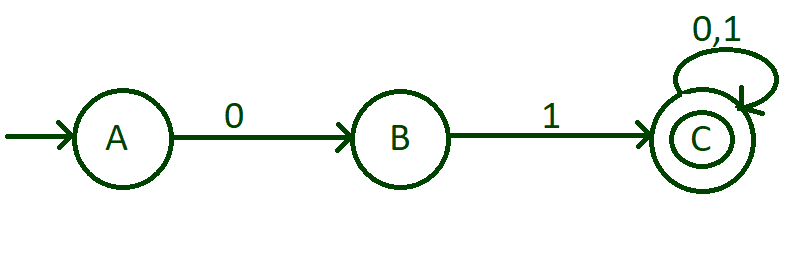• Step 3: Now, we need to think about the string that ends with “01”. We have done transition of 0 of state “A”, then with input 1 of the state “A”. With this minimum possible string that ends with 01 is 101. For this, do the transition of input 1 of state “A” to state “D” then do the transition of input 0 from the state “D” to state “E” and then do the transition of input 1 from sate “E” to state “F” and notice this “F” state as the final state.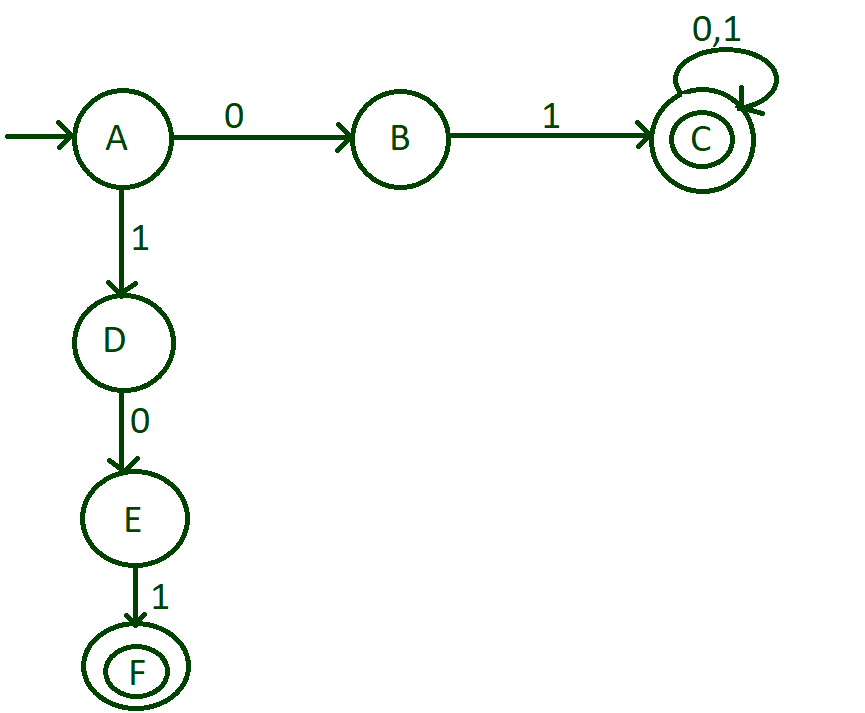• Step 4: There is one more possibility that any number of 1’s comes in beginning and then end with 01. For this, make a self-loop of 1 on state “D” and any number of zeros can come before 1 that comes in the end. For this, put a self-loop of 0 and state “E”.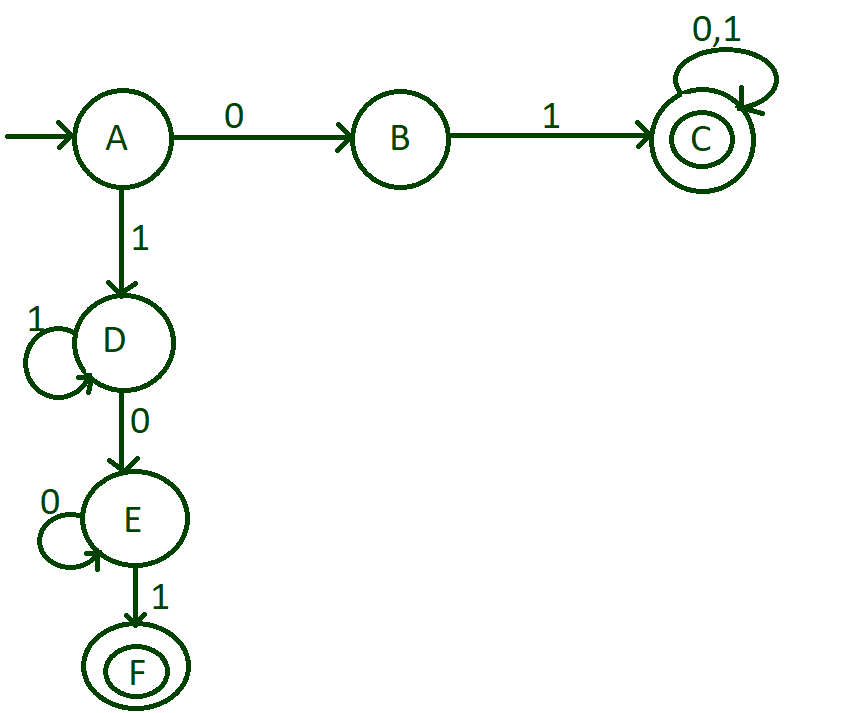• Step 5: Till now we have done with the strings that start with 1 and end with 01. Now, we need to think about the string that starts with 0 and ends with 01. For this, make a transition of input 0 from state “B” to state “E”.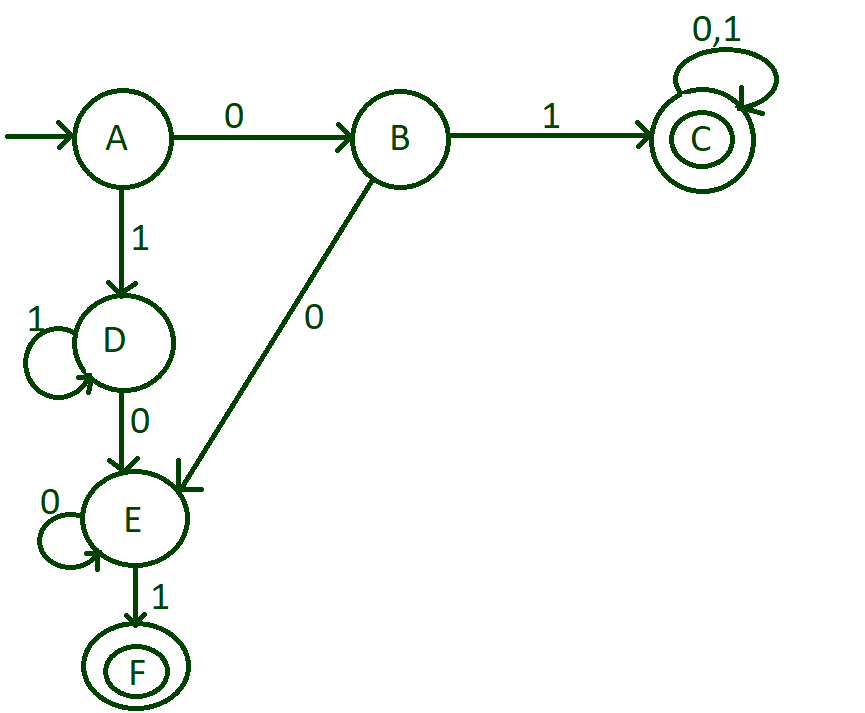• Step 6: Now we are just left with the input alphabets of the state “F”. Transit input 1 from state “F” to state “D” and then make transit input 0 from state “F” to state “E”.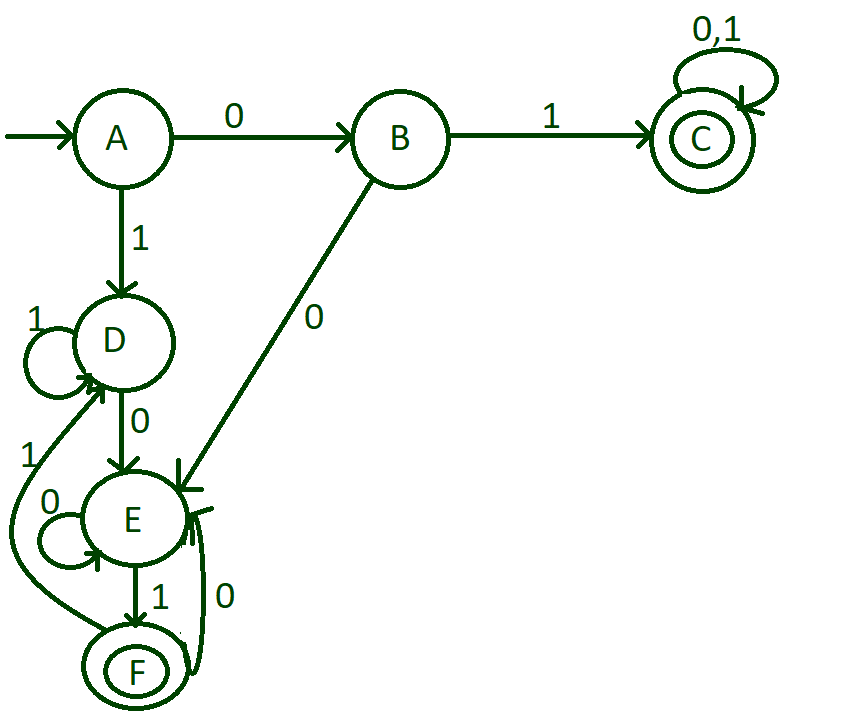Transition table and Transition rules of the above DFA: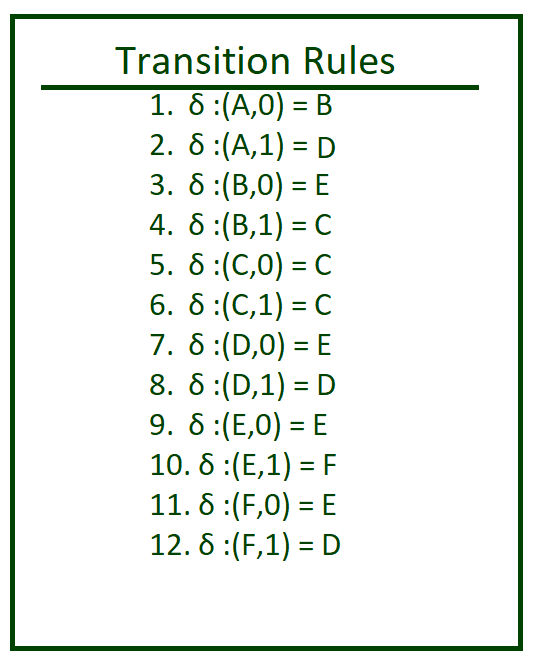Below is the implementation of the above approach:

## C++

 `// C++ program to check if a string ` `// either starts or ends with 01` `#include` `using` `namespace` `std;`   `void` `stateA(string);` `void` `stateB(string);` `void` `stateC(string);` `void` `stateD(string); ` `void` `stateE(string);` `void` `stateF(string);`   `// Function for transition ` `// state A` `void` `checkstateA(string n)` `{` `    `  `    ``// State transition to ` `    ``// B if the character is ` `    ``// 0` `    ``if``(n == ``'0'``)` `       ``stateB(n.substr(1));` `       `  `    ``// State transition to ` `    ``// D if the character is ` `    ``// 1` `    ``else` `       ``stateD(n.substr(1));` `}`   `// Function for transition ` `// state B         ` `void` `stateB(string n)` `{` `    `  `    ``// Check if the string has ` `    ``// ended` `    ``if` `(n.length() == 0)` `        ``cout << ``"string not accepted"``;` `    ``else` `    ``{` `        `  `        ``// State transition to C` `        ``// if the character is 1` `        ``if``(n == ``'1'``)` `            ``stateC(n.substr(1));`   `        ``// State transition to D` `        ``// if the character is 0` `        ``else` `            ``stateD(n.substr(1));` `    ``}     ` `} `   `// Function for transition ` `// state C ` `void` `stateC(string n)` `{` `    ``cout << ``"String accepted"``;` `}`   `// Function for transition ` `// state D` `void` `stateD(string n)` `{` `    ``if` `(n.length() == 0)` `        ``cout << ``"string not accepted"``;` `    ``else` `    ``{` `        `  `        ``// State transition to D` `        ``// if the character is 1` `        ``if` `(n == ``'1'``)` `            ``stateD(n.substr(1));`   `        ``// State transition to E` `        ``// if the character is 0` `        ``else` `            ``stateE(n.substr(1));` `    ``}` `}`   `// Function for transition ` `// state E` `void` `stateE(string n)` `{` `    ``if` `(n.length() == 0)` `        ``cout << ``"string not accepted"``;` `    ``else` `    ``{` `        `  `        ``// State transition to E` `        ``// if the character is 0 ` `        ``if``(n == ``'0'``)` `            ``stateE(n.substr(1));`   `        ``// State transition to F` `        ``// if the character is 1` `        ``else` `            ``stateF(n.substr(1));` `    ``}` `}`   `// Function for transition ` `// state F` `void` `stateF(string n)` `{` `    ``if``(n.length() == 0)` `        ``cout << ``"string accepred"``;` `    ``else` `    ``{` `        `  `        ``// State transition to D` `        ``// if the character is 1` `        ``if``(n == ``'1'``)` `            ``stateD(n.substr(1));`   `        ``// State transition to E` `        ``// if the character is 0` `        ``else` `            ``stateE(n.substr(1));` `    ``}` `} `   `// Driver code` `int` `main()` `{` `    ``string n = ``"0100101"``;` `    `  `    ``checkstateA(n);` `    ``return` `0;` `}`   `// This code is contributed by chitranayal`

## Java

 `// Java program to check if a string ` `// either starts or ends with 01` `import` `java.util.*; `   `class` `GFG{ ` `    `  `// Function for transition ` `// state A` `static` `void` `checkstateA(String n)` `{` `    `  `    ``// State transition to ` `    ``// B if the character is ` `    ``// 0` `    ``if` `(n.charAt(``0``) == ``'0'``)` `       ``stateB(n.substring(``1``));` `       `  `    ``// State transition to ` `    ``// D if the character is ` `    ``// 1` `    ``else` `       ``stateD(n.substring(``1``));` `}`   `// Function for transition ` `// state B         ` `static` `void` `stateB(String n)` `{` `    `  `    ``// Check if the string has ` `    ``// ended` `    ``if` `(n.length() == ``0``)` `        ``System.out.println(``"string not accepted"``);` `    ``else` `    ``{` `        `  `        ``// State transition to C` `        ``// if the character is 1` `        ``if` `(n.charAt(``0``) == ``'1'``)` `            ``stateC(n.substring(``1``));`   `        ``// State transition to D` `        ``// if the character is 0` `        ``else` `            ``stateD(n.substring(``1``));` `    ``}     ` `} `   `// Function for transition ` `// state C ` `static` `void` `stateC(String n)` `{` `    ``System.out.println(``"String accepted"``);` `}`   `// Function for transition ` `// state D` `static` `void` `stateD(String n)` `{` `    ``if` `(n.length() == ``0``)` `        ``System.out.println(``"string not accepted"``);` `    ``else` `    ``{` `        `  `        ``// State transition to D` `        ``// if the character is 1` `        ``if` `(n.charAt(``0``) == ``'1'``)` `            ``stateD(n.substring(``1``));`   `        ``// State transition to E` `        ``// if the character is 0` `        ``else` `            ``stateE(n.substring(``1``));` `    ``}` `}`   `// Function for transition ` `// state E` `static` `void` `stateE(String n)` `{` `    ``if` `(n.length() == ``0``)` `       ``System.out.println(``"string not accepted"``);` `    ``else` `    ``{` `        `  `        ``// State transition to E` `        ``// if the character is 0 ` `        ``if``(n.charAt(``0``) == ``'0'``)` `            ``stateE(n.substring(``1``));`   `        ``// State transition to F` `        ``// if the character is 1` `        ``else` `            ``stateF(n.substring(``1``));` `    ``}` `}`   `// Function for transition ` `// state F` `static` `void` `stateF(String n)` `{` `    ``if` `(n.length() == ``0``)` `        ``System.out.println(``"string accepred"``);` `    ``else` `    ``{` `        `  `        ``// State transition to D` `        ``// if the character is 1` `        ``if` `(n.charAt(``0``) == ``'1'``)` `            ``stateD(n.substring(``1``));`   `        ``// State transition to E` `        ``// if the character is 0` `        ``else` `            ``stateE(n.substring(``1``));` `    ``}` `} `   `// Driver Code` `public` `static` `void` `main(String args[])` `{` `    ``String n = ``"0100101"``;` `    `  `    ``checkstateA(n);` `}` `}`   `// This code is contributed by jyoti369`

## Python3

 `# Python3 program to check if ` `# a string either starts or` `# ends with 01`   `# Function for transition ` `# state A` `def` `checkstateA(n):`   `    ``# State transition to ` `    ``# B if the character is ` `    ``# 0` `    ``if``(n[``0``]``=``=``'0'``):` `        ``stateB(n[``1``:])`   `    ``# State transition to ` `    ``# D if the character is ` `    ``# 1` `    ``else``:` `        ``stateD(n[``1``:])`   `# Function for transition ` `# state B         ` `def` `stateB(n):`   `    ``# Check if the string has ` `    ``# ended` `    ``if` `(``len``(n)``=``=` `0``):` `        ``print``(``"string not accepted"``)` `    ``else``:    ` `    `  `        ``# State transition to C` `        ``# if the character is 1` `        ``if``(n[``0``]``=``=``'1'``):` `            ``stateC(n[``1``:])`   `        ``# State transition to D` `        ``# if the character is 0` `        ``else``:` `            ``stateD(n[``1``:])` `         `  `# Function for transition ` `# state C    ` `def` `stateC(n):` `    ``print``(``"String accepted"``)` ` `  `# Function for transition ` `# state D` `def` `stateD(n):` `    ``if` `(``len``(n)``=``=` `0``):` `        ``print``(``"string not accepted"``)` `    ``else``:    `   `        ``# State transition to D` `        ``# if the character is 1` `        ``if` `(n[``0``]``=``=``'1'``):` `            ``stateD(n[``1``:])`   `        ``# State transition to E` `        ``# if the character is 0` `        ``else``:` `            ``stateE(n[``1``:])` ` `  `# Function for transition ` `# state E` `def` `stateE(n):` `    ``if` `(``len``(n)``=``=` `0``):` `        ``print``(``"string not accepted"``)` `    ``else``:   `   `        ``# State transition to E` `        ``# if the character is 0 ` `        ``if``(n[``0``]``=``=``'0'``):` `            ``stateE(n[``1``:])`   `        ``# State transition to F` `        ``# if the character is 1` `        ``else``:` `            ``stateF(n[``1``:])` ` `  `# Function for transition ` `# state F` `def` `stateF(n):` `    ``if``(``len``(n)``=``=` `0``):` `        ``print``(``"string accepred"``)` `    ``else``:`   `        ``# State transition to D` `        ``# if the character is 1` `        ``if``(n[``0``]``=``=``'1'``):` `            ``stateD(n[``1``:])`   `        ``# State transition to E` `        ``# if the character is 0` `        ``else``:` `            ``stateE(n[``1``:])` `     `  `# Driver code` `if` `__name__ ``=``=` `"__main__"``:` `    ``n ``=` `"0100101"` `    ``checkstateA(n)`

## C#

 `// C# program to check if ` `// a string either starts ` `// or ends with 01` `using` `System;` `using` `System.Collections;` `using` `System.Collections.Generic;` `class` `GFG{` `    `  `// Function for transition ` `// state A` `static` `void` `checkstateA(``string` `n)` `{` `  ``// State transition to ` `  ``// B if the character is ` `  ``// 0` `  ``if``(n == ``'0'``)` `    ``stateB(n.Substring(1));`   `  ``// State transition to ` `  ``// D if the character is ` `  ``// 1` `  ``else` `    ``stateD(n.Substring(1));` `}` ` `  `// Function for transition ` `// state B         ` `static` `void` `stateB(``string` `n)` `{     ` `  ``// Check if the string has ` `  ``// ended` `  ``if` `(n.Length == 0)` `  ``{` `    ``Console.Write(``"string not accepted"``);` `  ``}` `  ``else` `  ``{` `    ``// State transition to C` `    ``// if the character is 1` `    ``if``(n == ``'1'``)` `      ``stateC(n.Substring(1));`   `    ``// State transition to D` `    ``// if the character is 0` `    ``else` `      ``stateD(n.Substring(1));` `  ``}     ` `} `   `// Function for transition ` `// state C ` `static` `void` `stateC(``string` `n)` `{` `  ``Console.Write(``"string accepted"``);` `}` ` `  `// Function for transition ` `// state D` `static` `void` `stateD(``string` `n)` `{` `  ``if` `(n.Length == 0)` `    ``Console.Write(``"string not accepted"``);` `  ``else` `  ``{`   `    ``// State transition to D` `    ``// if the character is 1` `    ``if` `(n == ``'1'``)` `      ``stateD(n.Substring(1));`   `    ``// State transition to E` `    ``// if the character is 0` `    ``else` `      ``stateE(n.Substring(1));` `  ``}` `}` ` `  `// Function for transition ` `// state E` `static` `void` `stateE(``string` `n)` `{` `  ``if` `(n.Length == 0)` `    ``Console.Write(``"string not accepted"``);` `  ``else` `  ``{`   `    ``// State transition to E` `    ``// if the character is 0 ` `    ``if``(n == ``'0'``)` `      ``stateE(n.Substring(1));`   `    ``// State transition to F` `    ``// if the character is 1` `    ``else` `      ``stateF(n.Substring(1));` `  ``}` `}` ` `  `// Function for transition ` `// state F` `static` `void` `stateF(``string` `n)` `{` `  ``if``(n.Length == 0)` `    ``Console.Write(``"string accepted"``);` `  ``else` `  ``{` `    ``// State transition to D` `    ``// if the character is 1` `    ``if``(n == ``'1'``)` `      ``stateD(n.Substring(1));`   `    ``// State transition to E` `    ``// if the character is 0` `    ``else` `      ``stateE(n.Substring(1));` `  ``}` `} `   `// Driver code` `public` `static` `void` `Main(``string` `[]args)` `{` `  ``string` `n = ``"0100101"``;` `  ``checkstateA(n);` `}` `}`   `// This code is contributed by rutvik_56`

Output:

```String accepted

```

Attention reader! Don’t stop learning now. Get hold of all the important DSA concepts with the DSA Self Paced Course at a student-friendly price and become industry ready.

My Personal Notes arrow_drop_up
Recommended Articles
Page :